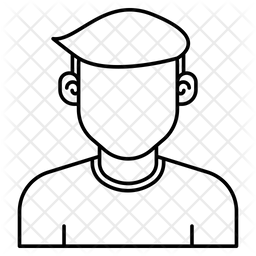607 viewsHello,

I want to calculate power for the 65th model of Dr. Hayes. I used Hayes'symbols. I added following interactions to "Power parameter section":

a1b3: = a1 * b3

a1b4: = a1 * b4

a3b1: = a3 * b1

a3b3: = a3 * b3

a3b4: = a3 * b4

I also added covariance to among the independent variables in the model, described all paths and covariances as "? .10".

Webpower gives a calculation error. What could be the source of the problem?

Thank you.by johnny (3.3k points)